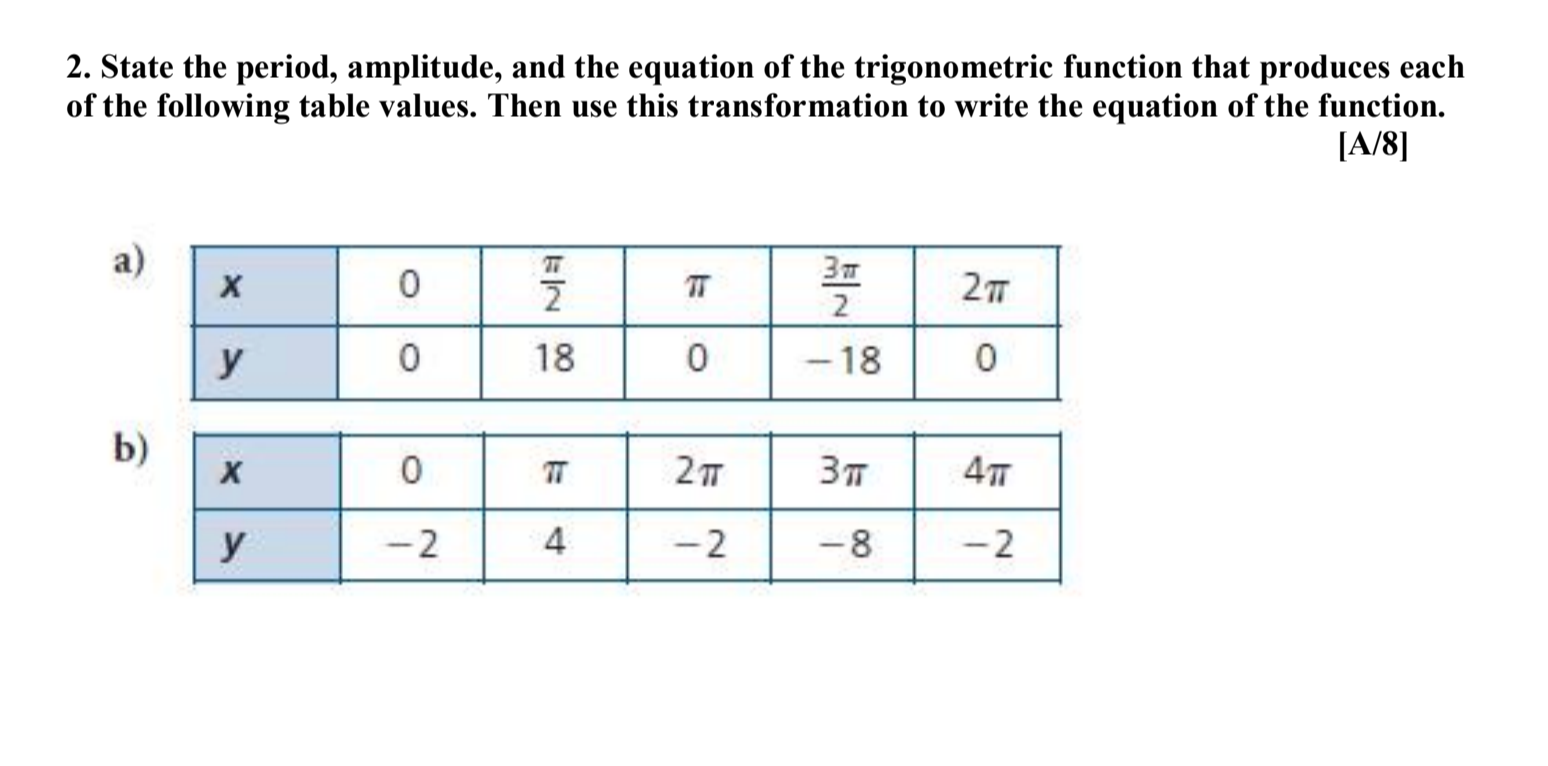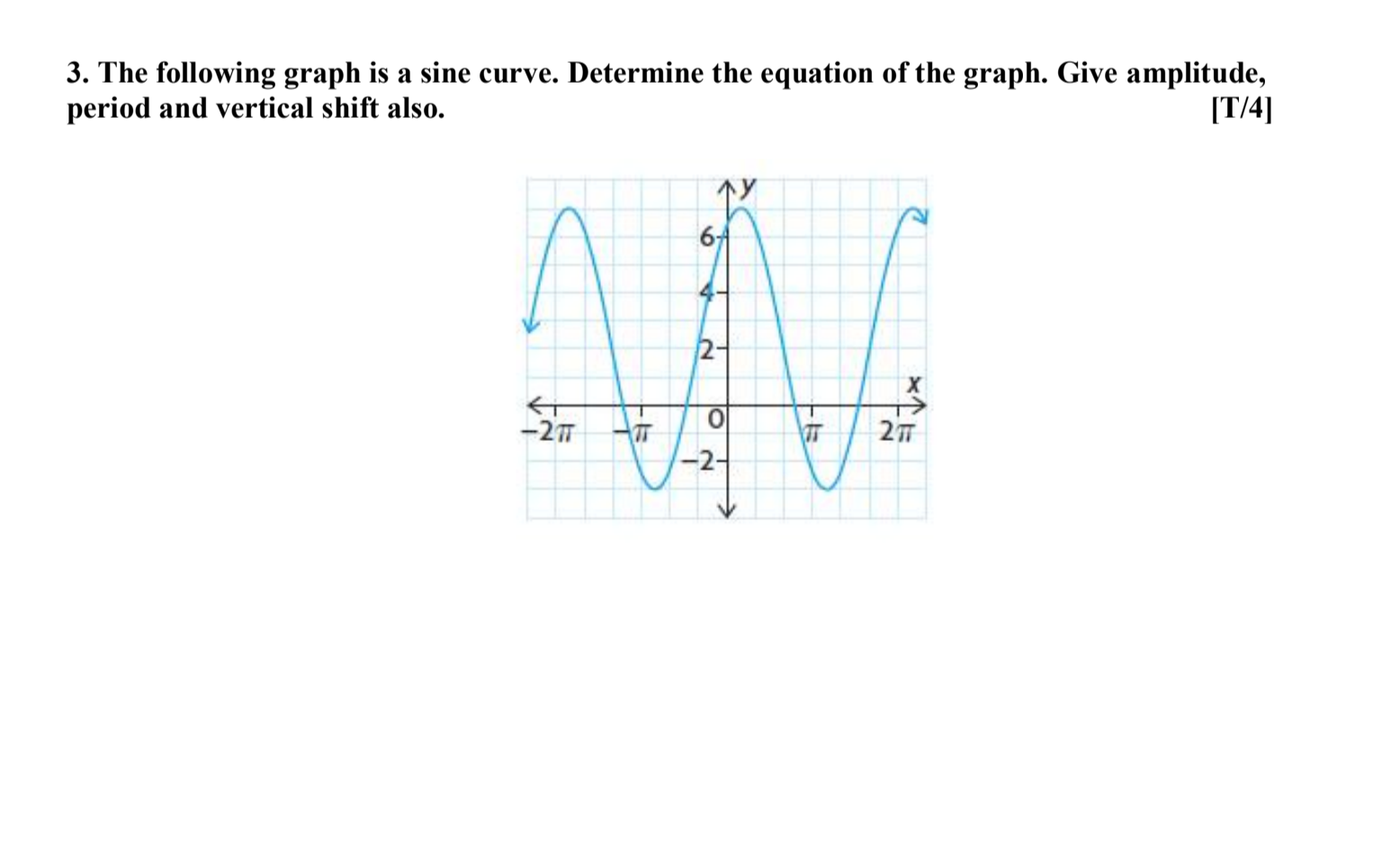# Question Solved1 Answer2. State the period, amplitude, and the equation of the trigonometric function that produces each of the following table values. Then use this transformation to write the equation of the function. [A/8] a) 0 2 37 3 2 2T y 0 18 0 -18 0 b) х 0 27 37 у 2 4 2 -8 -2 3. The following graph is a sine curve. Determine the equation of the graph. Give amplitude, period and vertical shift also. [T/4] 2- -27 277 -2Transcribed Image Text: 2. State the period, amplitude, and the equation of the trigonometric function that produces each of the following table values. Then use this transformation to write the equation of the function. [A/8] a) 0 2 37 3 2 2T y 0 18 0 -18 0 b) х 0 27 37 у 2 4 2 -8 -2 3. The following graph is a sine curve. Determine the equation of the graph. Give amplitude, period and vertical shift also. [T/4] 2- -27 277 -2
More
Transcribed Image Text: 2. State the period, amplitude, and the equation of the trigonometric function that produces each of the following table values. Then use this transformation to write the equation of the function. [A/8] a) 0 2 37 3 2 2T y 0 18 0 -18 0 b) х 0 27 37 у 2 4 2 -8 -2 3. The following graph is a sine curve. Determine the equation of the graph. Give amplitude, period and vertical shift also. [T/4] 2- -27 277 -2